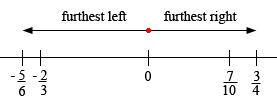SEARCH HOMEMath Central Quandaries & QueriesQuestion from Christyrose, a student: Hello. I am a sixth grade math student and i need help on how to compare negative and positive fractions. Here is is: 3/4 -2/3 7/10 -5/6 I'm not sure how to do the negative parts because i was absent for a couple days. Thank you! ChristyroseHi Christyrose,

Think about putting these fractions on the number line.

Look at the positive values first and express them with a common denominator

3/4 = 15/20 and 7/10 = 14/20.

Now you can see clearly that 14/20 < 15/20 so 7/10 < 3/4. On the number line this means that 7/10 is closer to zero than 3/4, both to the right of zero.

Now look at the negative values and, for the moment ignore the negative signs. Again find a common denominator.

2/3 = 4/6 and compare to 5/6.

Since 4/6 < 5/6 we know that 4/6 = 2/3 is closer to zero than 5/6. But the negative signs in front of -2/3 and -5/6 tell us that these numbers are to the left of zero.

Thus, from left to right the furthest from zero is -5/6, next is -2/3 then we pass zero and the closest to zero is 7/10 and finally 3/4.I hope this helps,
PennyMath Central is supported by the University of Regina and The Pacific Institute for the Mathematical Sciences.